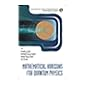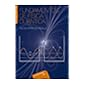Normal view MARC view ISBD view

# Quantum mechanics : two volumes bound as one / Albert Messiah.

Material type:BookSeries: Dover books on physics.Copyright date: Minneola, NY : Dover , 2014Edition: First Edition.Description: xxi, 1136 pages : illustrations ; 22 cm.ISBN: 9780486784557.DDC classification: 530.12
Partial contents:
Contents of Volume I -- Part 1. The formalism and its Interpretation -- Chapter 1. The origins of the quantum theory -- Chapter 2. Matter Waves and the Schrodinger Equation -- Chapter 3. One-Dimensional Quantized Systems -- Chapter 4. Statistical Interpretation of the wave-corpuscle duality and the uncertainty relations -- Chapter 5. Development of the formalism of wave mechanics and its interpretation -- Chapter 6. Classical Approximation and the WKB Method -- Chapter 7. General Formalism of the quantum theory: (A) Mathematical framework -- Chapter 8. General Formalism: (B) Description of physical phenomena -- Part 2. Simple Systems -- Chapter 9. Solution of the Schrodinger Equation by Separation of variables. Central potential -- Chapter 10. Scattering problems. Central potential and phase-shift method -- Chapter 11. The Coulomb interaction -- Chapter 12. The harmonic oscillator -- Contents of Volume II -- Part 3. Symmetries and Invariance -- Chapter 13. Angular momentum in quantum mechanics -- Chapter 14. Systems of identical particles. Pauli exclusion principle -- Chapter 15. Invariance and conservation theorems. Time reversal -- Part 4. Methods of Approximation -- Chapter 16. Stationary Perturbations -- Chapter 17. Approximate solutions of the time-dependent Schrodinger equation -- Chapter 18. The variational method and associated problems -- Chapter 19. Collision theory -- Part 5. Elements of Relativistic quantum mechanics -- Chapter 20. The Dirac equation -- Chapter 21. Field quantization. Radiation theory.
Abstract: &quot;Strongly recommended&quot; by the American Journal of Physics, this volume serves as a text for advanced undergraduates and graduate students of physics as well as a reference for professionals. Clear in its presentation and scrupulous in its attention to detail, the treatment originally appeared in a two-volume French edition. This convenient single-volume translation begins with formalism and its interpretation, starting with the origins of quantum theory and examinations of matter waves and the Schrd̲inger equation, one-dimensional quantized systems, the uncertainty relations, and the mathematical framework and physical content of formalism. The second half opens with an exploration of symmetries and invariance, including a consideration of angular momentum, identical particles and the Pauli exclusion principle, invariance and conservation laws, and time reversal. Methods of approximation include those involving stationary perturbations, the equation of motion, variational method, and collision theory. The final chapters review the elements of relativistic quantum mechanics, and each of the two volumes concludes with useful appendixes.
Tags from this library: No tags from this library for this title.
Item type Current location Call number Copy number Status Date due Item holdsLibro académico
530.12 M5851q 2014 (Browse shelf) Ej. 1 Available
Total holds: 0
##### Browsing Biblioteca del Campus Shelves Close shelf browser530.12 M4263 2010 Mathematical horizons for quantum physics / 530.12 M4263 2010 Mathematical horizons for quantum physics / 530.12 M4263 2010 Mathematical horizons for quantum physics / 530.12 M5851q 2014 Quantum mechanics : 530.12 P4379f 2011 Fundamentos de física cuántica / 530.12 P4379f 2011 Fundamentos de física cuántica / 530.12 P4379f 2011 Fundamentos de física cuántica /

Reprint de la traducción inglesa de Mecanique cuantique, 1961-62.

Contents of Volume I -- Part 1. The formalism and its Interpretation -- Chapter 1. The origins of the quantum theory -- Chapter 2. Matter Waves and the Schrodinger Equation -- Chapter 3. One-Dimensional Quantized Systems -- Chapter 4. Statistical Interpretation of the wave-corpuscle duality and the uncertainty relations -- Chapter 5. Development of the formalism of wave mechanics and its interpretation -- Chapter 6. Classical Approximation and the WKB Method -- Chapter 7. General Formalism of the quantum theory: (A) Mathematical framework -- Chapter 8. General Formalism: (B) Description of physical phenomena -- Part 2. Simple Systems -- Chapter 9. Solution of the Schrodinger Equation by Separation of variables. Central potential -- Chapter 10. Scattering problems. Central potential and phase-shift method -- Chapter 11. The Coulomb interaction -- Chapter 12. The harmonic oscillator -- Contents of Volume II -- Part 3. Symmetries and Invariance -- Chapter 13. Angular momentum in quantum mechanics -- Chapter 14. Systems of identical particles. Pauli exclusion principle -- Chapter 15. Invariance and conservation theorems. Time reversal -- Part 4. Methods of Approximation -- Chapter 16. Stationary Perturbations -- Chapter 17. Approximate solutions of the time-dependent Schrodinger equation -- Chapter 18. The variational method and associated problems -- Chapter 19. Collision theory -- Part 5. Elements of Relativistic quantum mechanics -- Chapter 20. The Dirac equation -- Chapter 21. Field quantization. Radiation theory.

"Strongly recommended" by the American Journal of Physics, this volume serves as a text for advanced undergraduates and graduate students of physics as well as a reference for professionals. Clear in its presentation and scrupulous in its attention to detail, the treatment originally appeared in a two-volume French edition. This convenient single-volume translation begins with formalism and its interpretation, starting with the origins of quantum theory and examinations of matter waves and the Schrd̲inger equation, one-dimensional quantized systems, the uncertainty relations, and the mathematical framework and physical content of formalism. The second half opens with an exploration of symmetries and invariance, including a consideration of angular momentum, identical particles and the Pauli exclusion principle, invariance and conservation laws, and time reversal. Methods of approximation include those involving stationary perturbations, the equation of motion, variational method, and collision theory. The final chapters review the elements of relativistic quantum mechanics, and each of the two volumes concludes with useful appendixes.

There are no comments for this item.